# ML Aggarwal Solutions for Class 8 Maths Chapter 5 - Playing with Numbers

ML Aggarwal Solutions for Class 8 Maths Chapter 5 – Playing with Numbers are provided here to help students prepare for their exams at ease. This chapter mainly deals with problems based on numbers, which includes topics like generalized form of numbers, tests of divisibility, interchanging the digits numbers, etc. Students are provided with exercise-wise solutions to help understand the concepts clearly from the exam point of view. Our expert tutors have solved the problems using short cut methods, which help students in solving the problems at a good speed. Students aspiring to secure high marks in their examination are advised to practice the solutions on a regular basis. ML Aggarwal Solutions PDF can be downloaded from the links provided below and students can use it for future reference as well.

Chapter 5 – Playing with Numbers contains three exercises and the ML Aggarwal Class 8 Solutions present in this page provides solutions to questions related to each exercise present in this chapter.

## Download the Pdf of ML Aggarwal Solutions for Class 8 Maths Chapter 5 – Playing with Numbers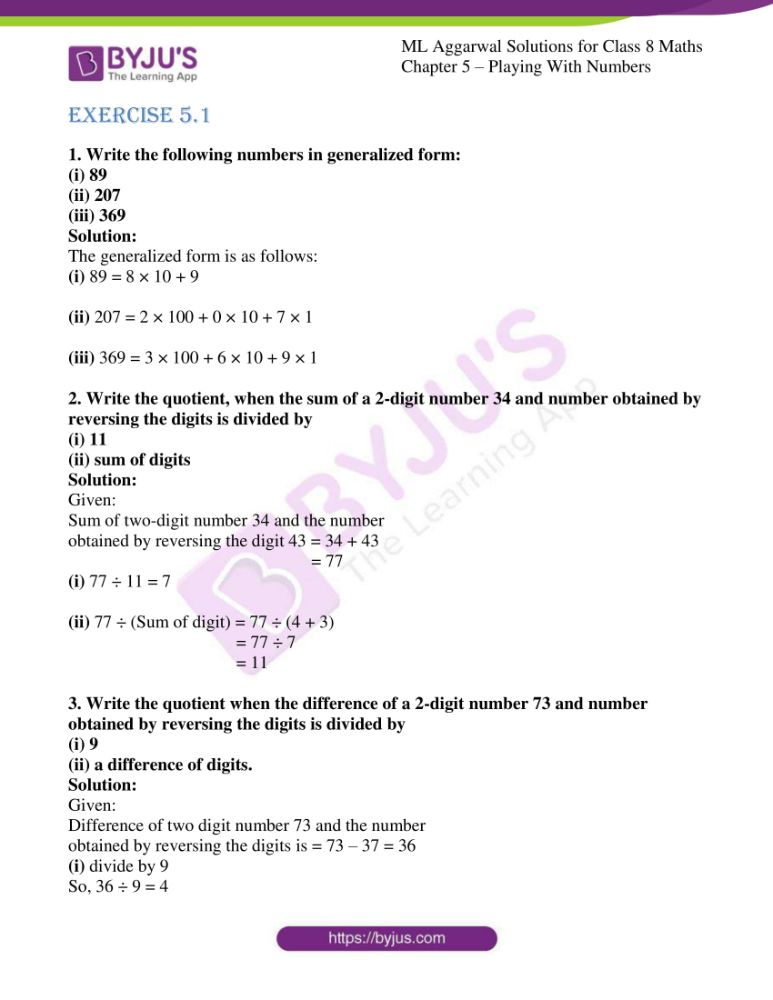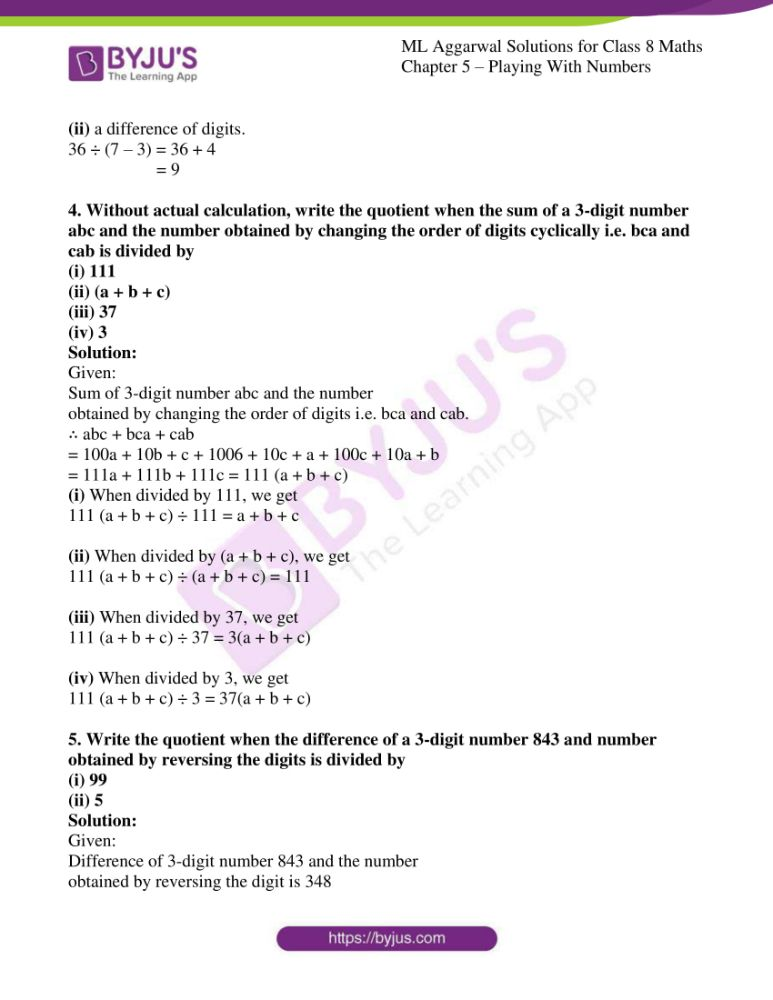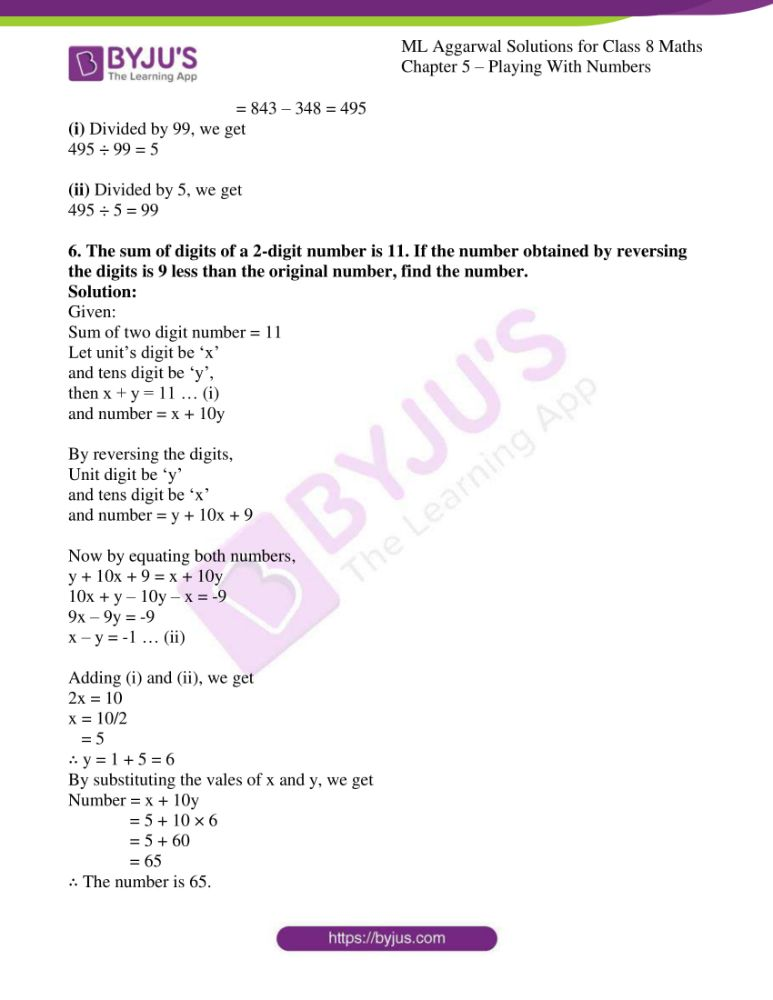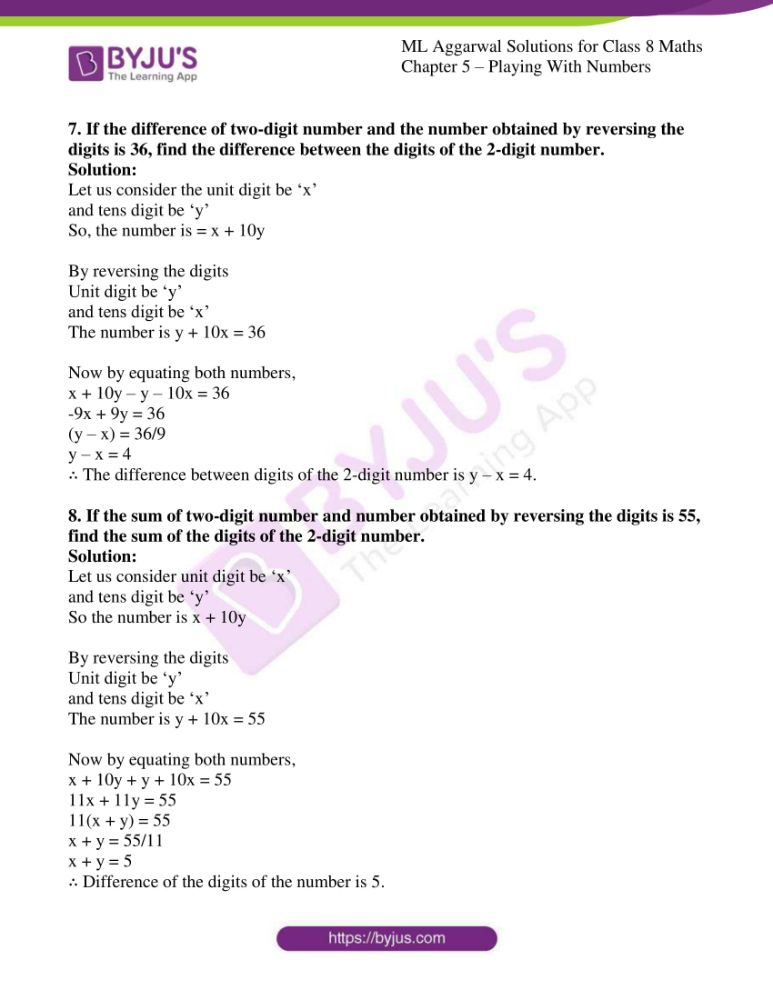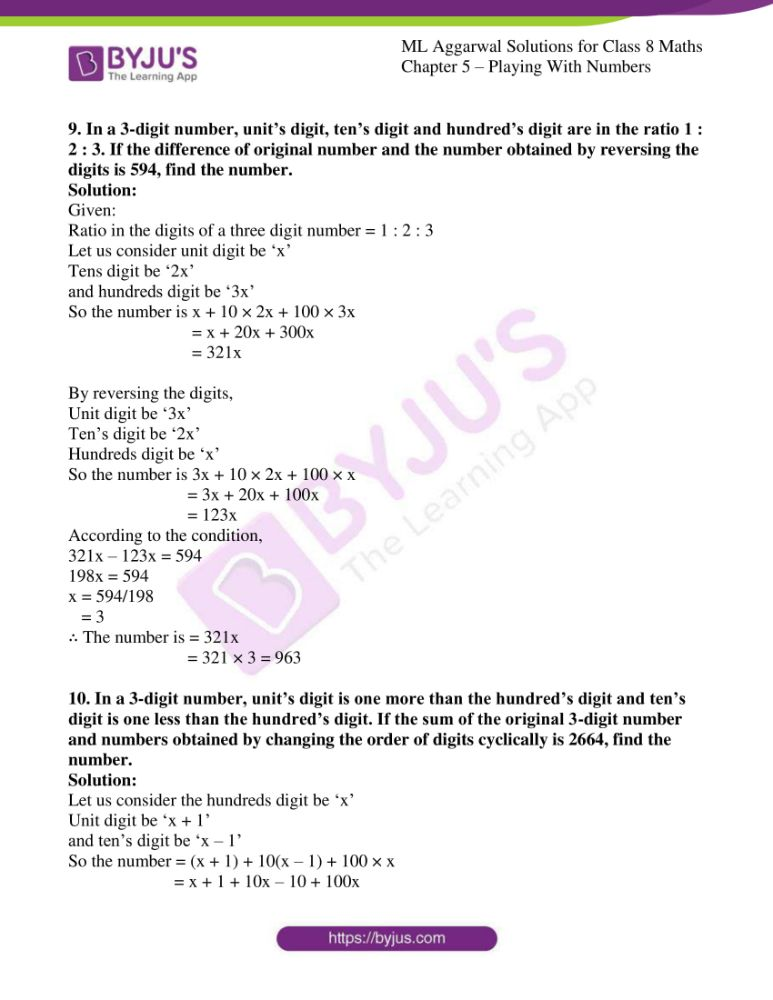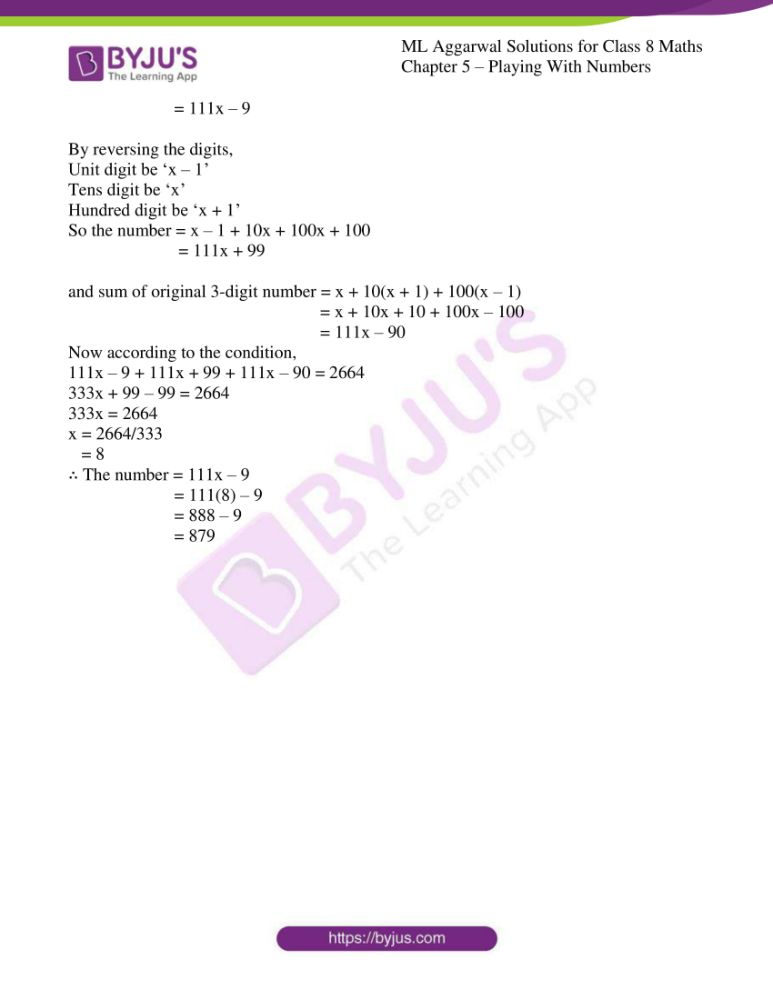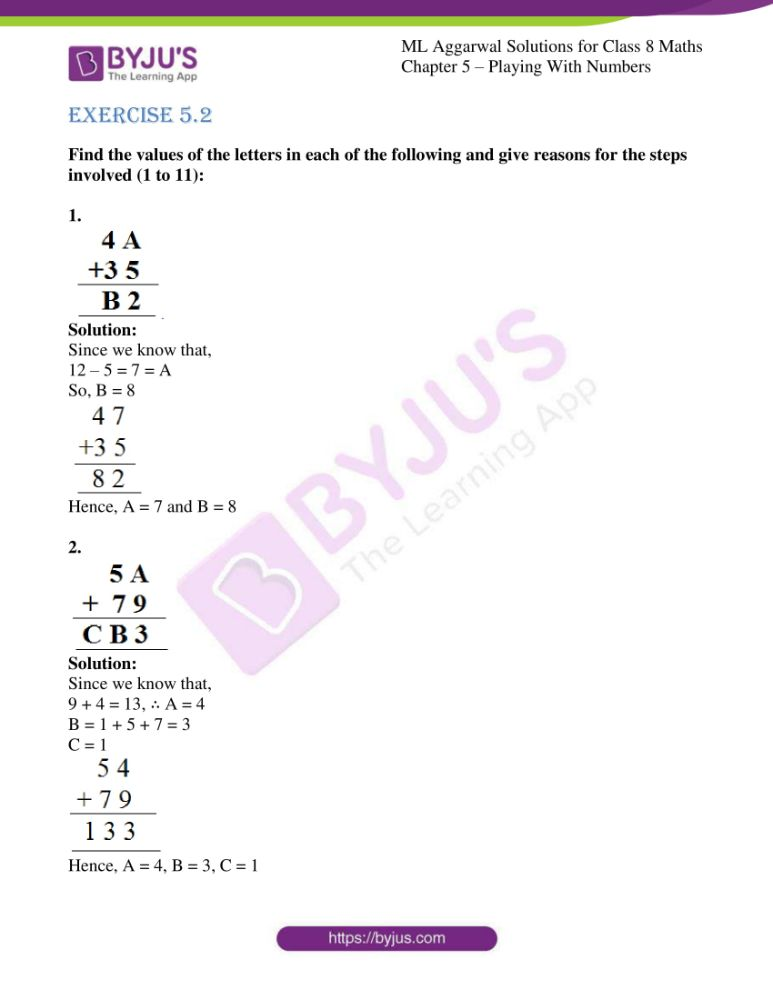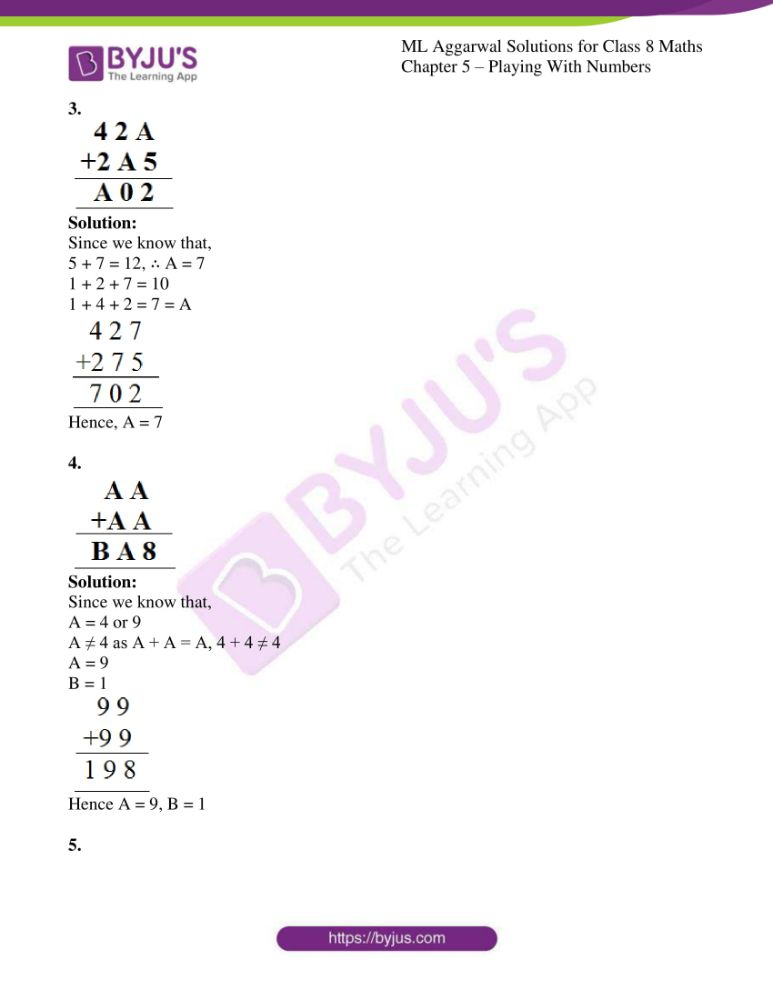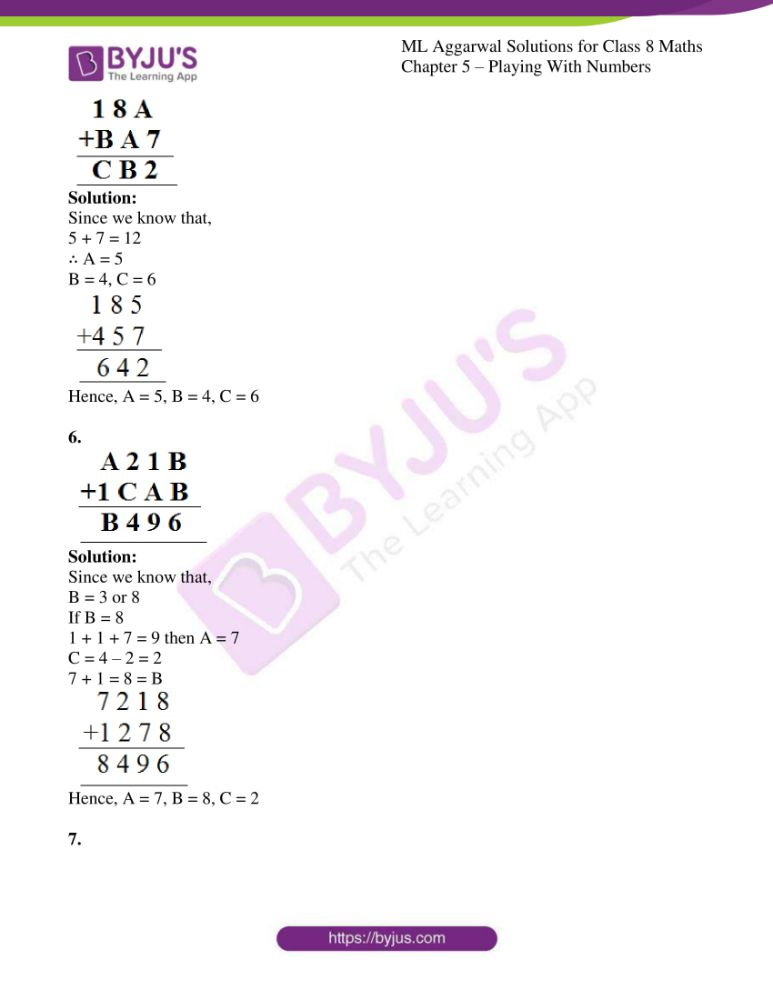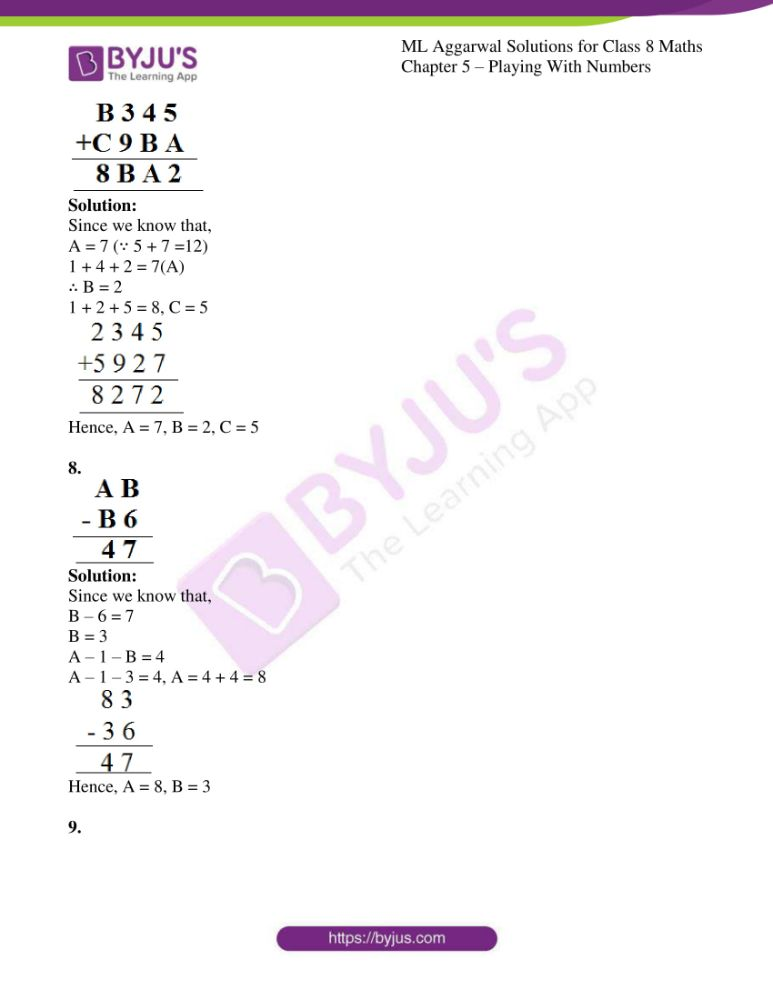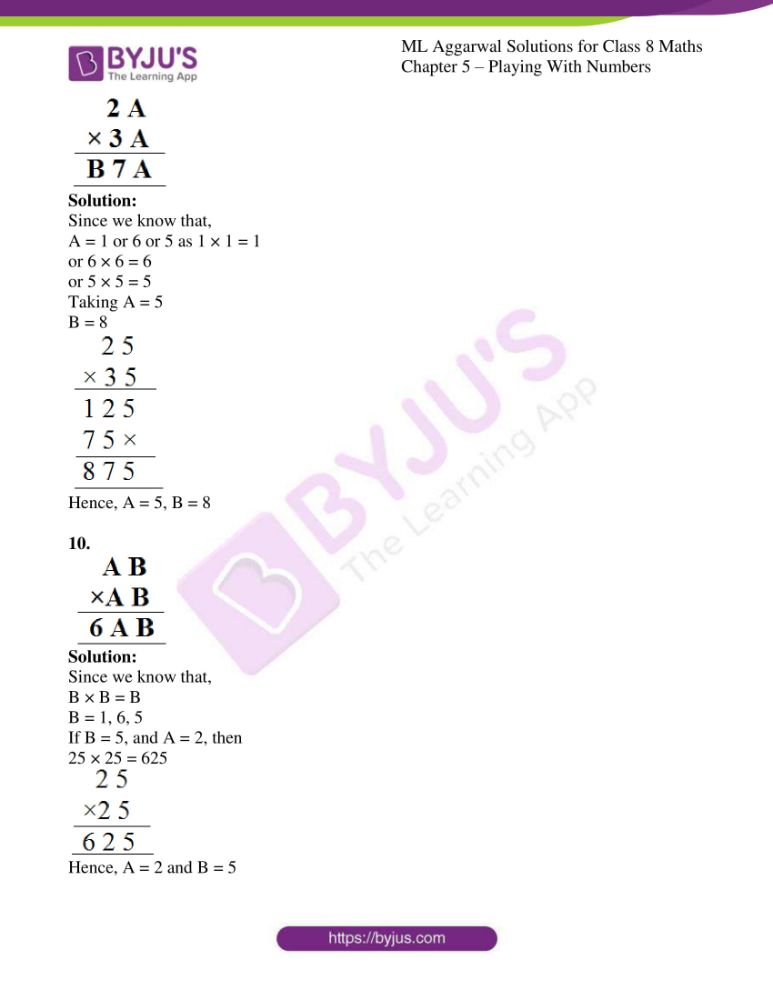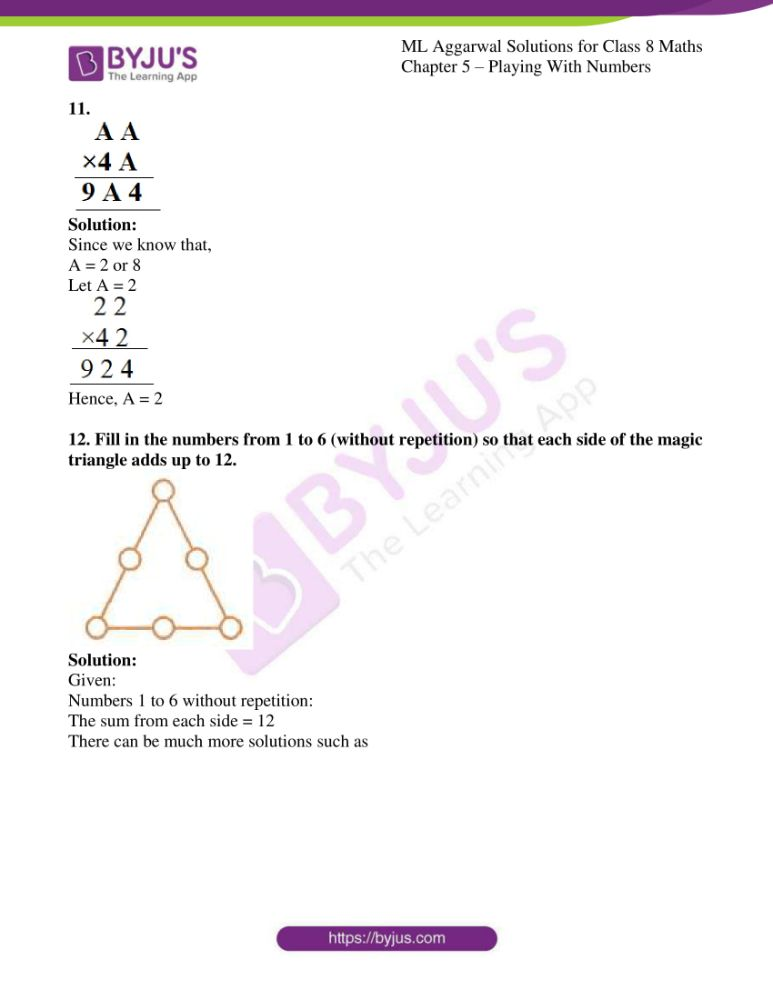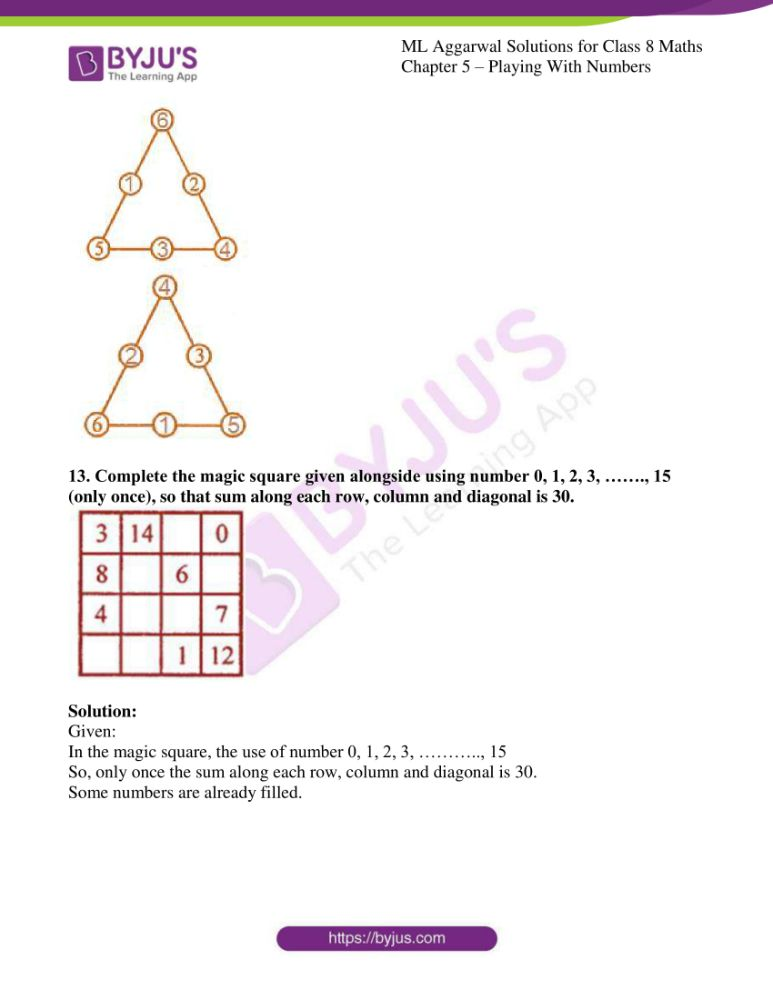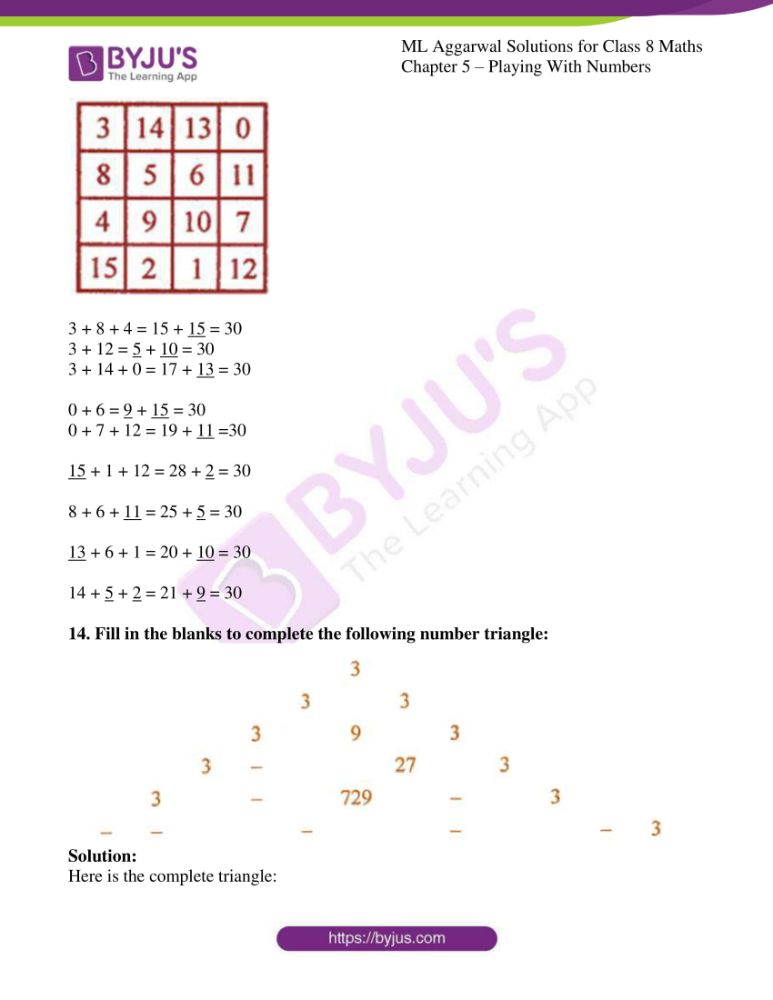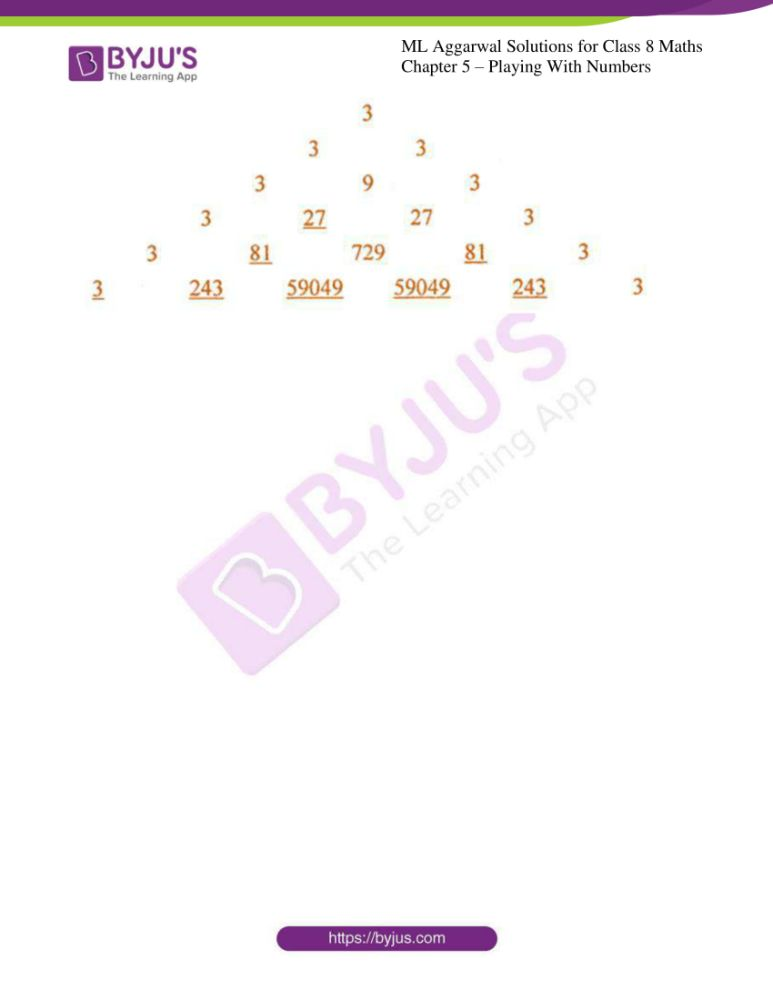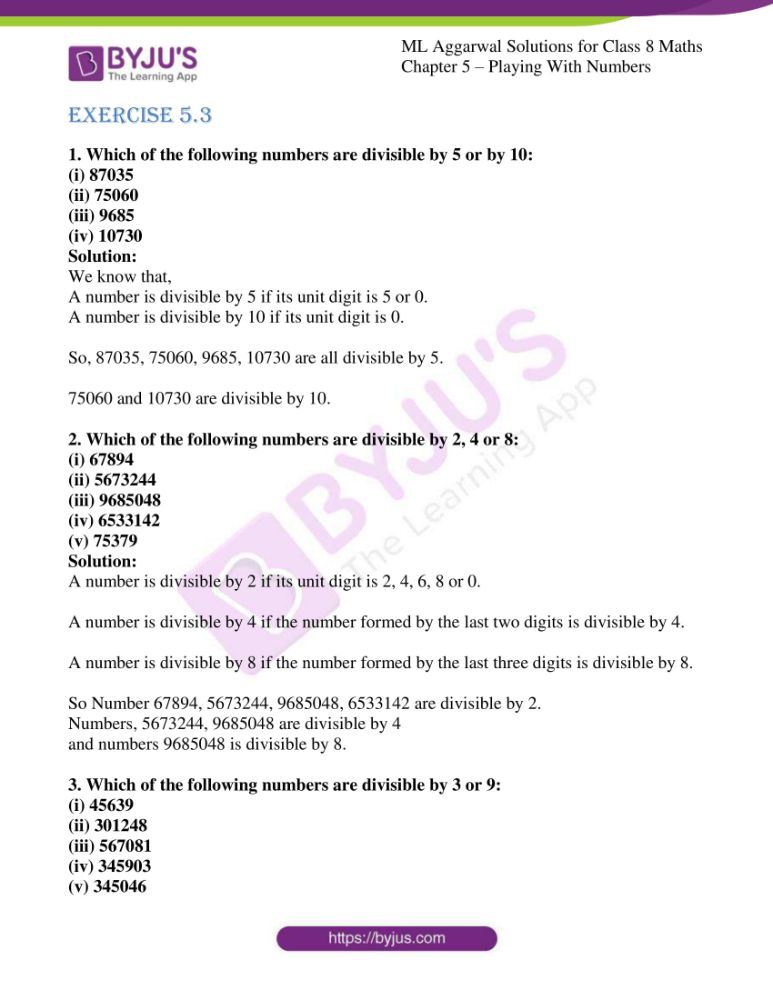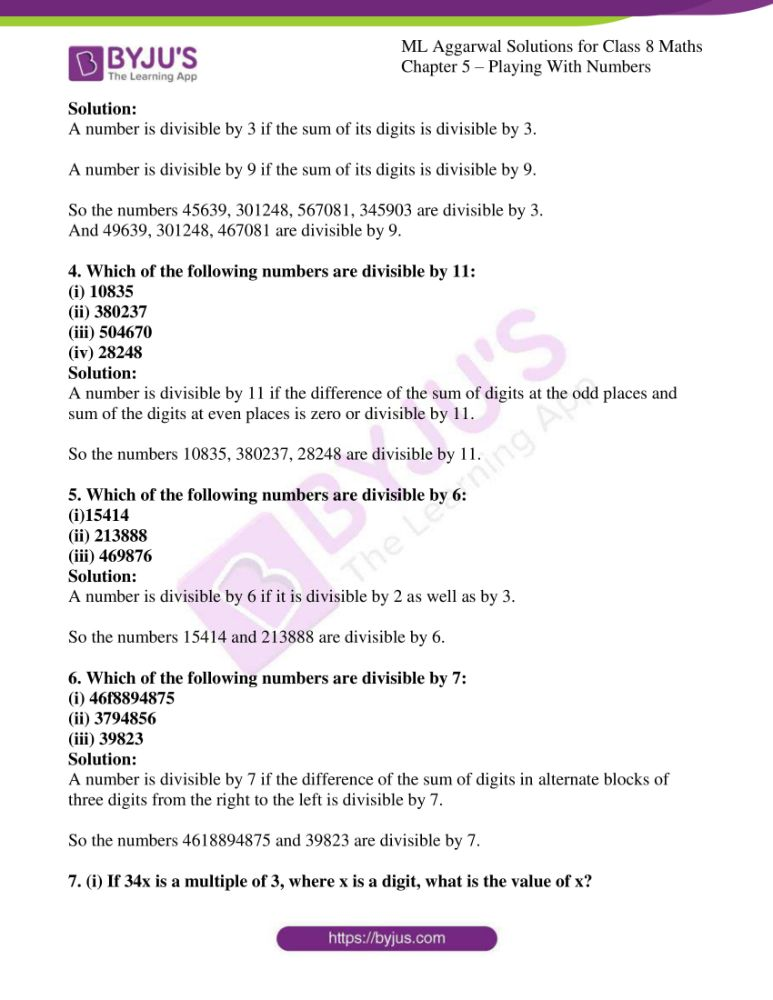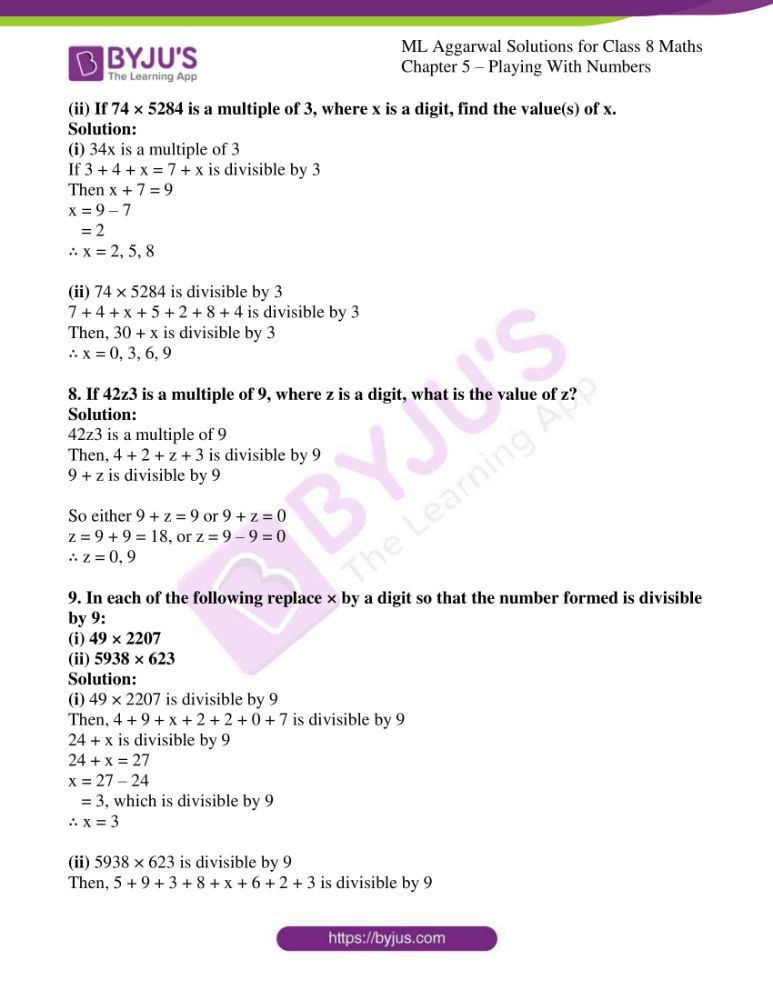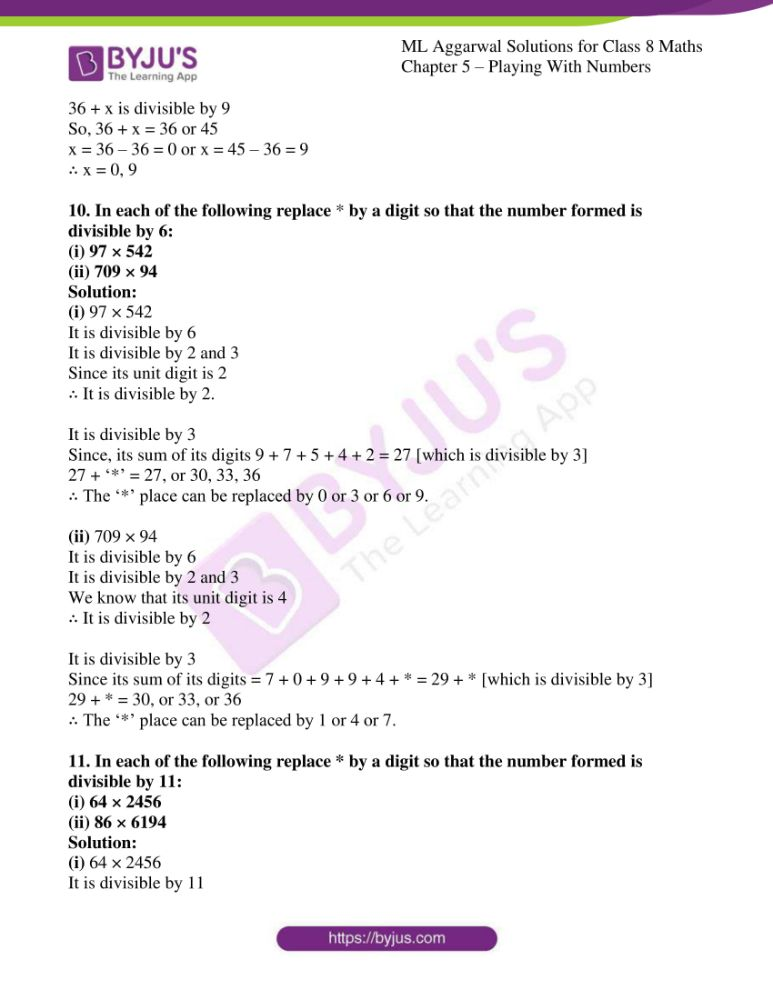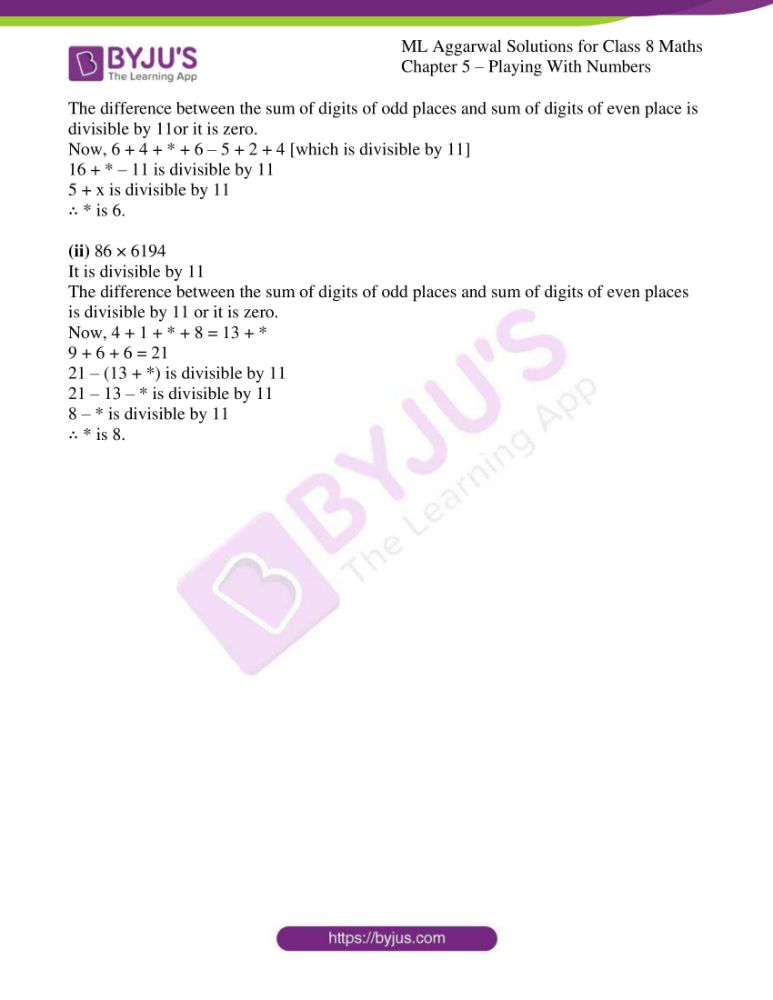### Access answers to ML Aggarwal Solutions for Class 8 Maths Chapter 5 – Playing with Numbers

EXERCISE 5.1

1. Write the following numbers in generalized form:
(i) 89
(ii) 207
(iii) 369
Solution:

The generalized form is as follows:

(i) 89 = 8 × 10 + 9

(ii) 207 = 2 × 100 + 0 × 10 + 7 × 1

(iii) 369 = 3 × 100 + 6 × 10 + 9 × 1

2. Write the quotient, when the sum of a 2-digit number 34 and number obtained by reversing the digits is divided by
(i) 11
(ii) sum of digits
Solution:

Given:

Sum of two-digit number 34 and the number

obtained by reversing the digit 43 = 34 + 43

= 77

(i) 77 ÷ 11 = 7

(ii) 77 ÷ (Sum of digit) = 77 ÷ (4 + 3)

= 77 ÷ 7

= 11

3. Write the quotient when the difference of a 2-digit number 73 and number obtained by reversing the digits is divided by
(i) 9
(ii) a difference of digits.
Solution:

Given:

Difference of two digit number 73 and the number

obtained by reversing the digits is = 73 – 37 = 36

(i) divide by 9

So, 36 ÷ 9 = 4

(ii) a difference of digits.

36 ÷ (7 – 3) = 36 + 4

= 9

4. Without actual calculation, write the quotient when the sum of a 3-digit number abc and the number obtained by changing the order of digits cyclically i.e. bca and cab is divided by
(i) 111
(ii) (a + b + c)
(iii) 37
(iv) 3
Solution:

Given:

Sum of 3-digit number abc and the number

obtained by changing the order of digits i.e. bca and cab.

∴ abc + bca + cab

= 100a + 10b + c + 1006 + 10c + a + 100c + 10a + b

= 111a + 111b + 111c = 111 (a + b + c)

(i) When divided by 111, we get

111 (a + b + c) ÷ 111 = a + b + c

(ii) When divided by (a + b + c), we get

111 (a + b + c) ÷ (a + b + c) = 111

(iii) When divided by 37, we get

111 (a + b + c) ÷ 37 = 3(a + b + c)

(iv) When divided by 3, we get

111 (a + b + c) ÷ 3 = 37(a + b + c)

5. Write the quotient when the difference of a 3-digit number 843 and number obtained by reversing the digits is divided by
(i) 99
(ii) 5
Solution:

Given:

Difference of 3-digit number 843 and the number

obtained by reversing the digit is 348

= 843 – 348 = 495

(i) Divided by 99, we get

495 ÷ 99 = 5

(ii) Divided by 5, we get

495 ÷ 5 = 99

6. The sum of digits of a 2-digit number is 11. If the number obtained by reversing the digits is 9 less than the original number, find the number.
Solution:

Given:

Sum of two digit number = 11

Let unit’s digit be ‘x’

and tens digit be ‘y’,

then x + y = 11 … (i)

and number = x + 10y

By reversing the digits,

Unit digit be ‘y’

and tens digit be ‘x’

and number = y + 10x + 9

Now by equating both numbers,

y + 10x + 9 = x + 10y

10x + y – 10y – x = -9

9x – 9y = -9

x – y = -1 … (ii)

Adding (i) and (ii), we get

2x = 10

x = 10/2

= 5

∴ y = 1 + 5 = 6

By substituting the vales of x and y, we get

Number = x + 10y

= 5 + 10 × 6

= 5 + 60

= 65

∴ The number is 65.

7. If the difference of two-digit number and the number obtained by reversing the digits is 36, find the difference between the digits of the 2-digit number.
Solution:

Let us consider the unit digit be ‘x’

and tens digit be ‘y’

So, the number is = x + 10y

By reversing the digits

Unit digit be ‘y’

and tens digit be ‘x’

The number is y + 10x = 36

Now by equating both numbers,

x + 10y – y – 10x = 36

-9x + 9y = 36

(y – x) = 36/9

y – x = 4

∴ The difference between digits of the 2-digit number is y – x = 4.

8. If the sum of two-digit number and number obtained by reversing the digits is 55, find the sum of the digits of the 2-digit number.
Solution:

Let us consider unit digit be ‘x’

and tens digit be ‘y’

So the number is x + 10y

By reversing the digits

Unit digit be ‘y’

and tens digit be ‘x’

The number is y + 10x = 55

Now by equating both numbers,

x + 10y + y + 10x = 55

11x + 11y = 55

11(x + y) = 55

x + y = 55/11

x + y = 5

∴ Difference of the digits of the number is 5.

9. In a 3-digit number, unit’s digit, ten’s digit and hundred’s digit are in the ratio 1 : 2 : 3. If the difference of original number and the number obtained by reversing the digits is 594, find the number.
Solution:

Given:

Ratio in the digits of a three digit number = 1 : 2 : 3

Let us consider unit digit be ‘x’

Tens digit be ‘2x’

and hundreds digit be ‘3x’

So the number is x + 10 × 2x + 100 × 3x

= x + 20x + 300x

= 321x

By reversing the digits,

Unit digit be ‘3x’

Ten’s digit be ‘2x’

Hundreds digit be ‘x’

So the number is 3x + 10 × 2x + 100 × x

= 3x + 20x + 100x

= 123x

According to the condition,

321x – 123x = 594

198x = 594

x = 594/198

= 3

∴ The number is = 321x

= 321 × 3 = 963

10. In a 3-digit number, unit’s digit is one more than the hundred’s digit and ten’s digit is one less than the hundred’s digit. If the sum of the original 3-digit number and numbers obtained by changing the order of digits cyclically is 2664, find the number.
Solution:

Let us consider the hundreds digit be ‘x’

Unit digit be ‘x + 1’

and ten’s digit be ‘x – 1’

So the number = (x + 1) + 10(x – 1) + 100 × x

= x + 1 + 10x – 10 + 100x

= 111x – 9

By reversing the digits,

Unit digit be ‘x – 1’

Tens digit be ‘x’

Hundred digit be ‘x + 1’

So the number = x – 1 + 10x + 100x + 100

= 111x + 99

and sum of original 3-digit number = x + 10(x + 1) + 100(x – 1)

= x + 10x + 10 + 100x – 100

= 111x – 90

Now according to the condition,

111x – 9 + 111x + 99 + 111x – 90 = 2664

333x + 99 – 99 = 2664

333x = 2664

x = 2664/333

= 8

∴ The number = 111x – 9

= 111(8) – 9

= 888 – 9

= 879

EXERCISE 5.2

Find the values of the letters in each of the following and give reasons for the steps involved (1 to 11):

1.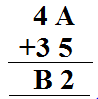Solution:

Since we know that,

12 – 5 = 7 = A

So, B = 8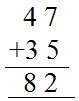Hence, A = 7 and B = 8

2.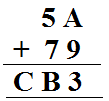Solution:

Since we know that,

9 + 4 = 13, ∴ A = 4

B = 1 + 5 + 7 = 3

C = 1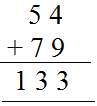Hence, A = 4, B = 3, C = 1

3.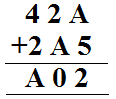Solution:

Since we know that,

5 + 7 = 12, ∴ A = 7

1 + 2 + 7 = 10

1 + 4 + 2 = 7 = A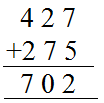Hence, A = 7

4.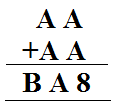Solution:

Since we know that,

A = 4 or 9

A ≠ 4 as A + A = A, 4 + 4 ≠ 4

A = 9

B = 1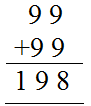Hence A = 9, B = 1

5.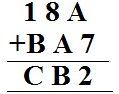Solution:

Since we know that,

5 + 7 = 12

∴ A = 5

B = 4, C = 6Hence, A = 5, B = 4, C = 6

6.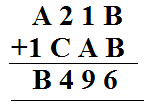Solution:

Since we know that,

B = 3 or 8

If B = 8

1 + 1 + 7 = 9 then A = 7

C = 4 – 2 = 2

7 + 1 = 8 = B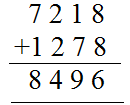Hence, A = 7, B = 8, C = 2

7.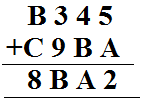Solution:

Since we know that,

A = 7 (∵ 5 + 7 =12)

1 + 4 + 2 = 7(A)

∴ B = 2

1 + 2 + 5 = 8, C = 5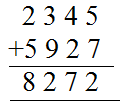Hence, A = 7, B = 2, C = 5

8.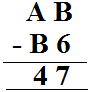Solution:

Since we know that,

B – 6 = 7

B = 3

A – 1 – B = 4

A – 1 – 3 = 4, A = 4 + 4 = 8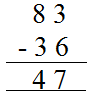Hence, A = 8, B = 3

9.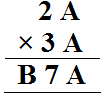Solution:

Since we know that,

A = 1 or 6 or 5 as 1 × 1 = 1

or 6 × 6 = 6

or 5 × 5 = 5

Taking A = 5

B = 8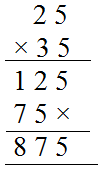Hence, A = 5, B = 8

10.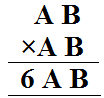Solution:

Since we know that,

B × B = B

B = 1, 6, 5

If B = 5, and A = 2, then

25 × 25 = 625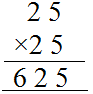Hence, A = 2 and B = 5

11.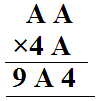Solution:

Since we know that,

A = 2 or 8

Let A = 2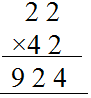Hence, A = 2

12. Fill in the numbers from 1 to 6 (without repetition) so that each side of the magic triangle adds up to 12.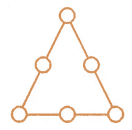Solution:

Given:

Numbers 1 to 6 without repetition:

The sum from each side = 12

There can be much more solutions such as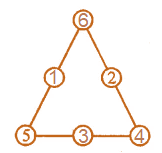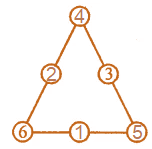13. Complete the magic square given alongside using number 0, 1, 2, 3, ……., 15 (only once), so that sum along each row, column and diagonal is 30.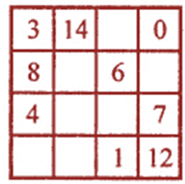Solution:

Given:

In the magic square, the use of number 0, 1, 2, 3, ……….., 15

So, only once the sum along each row, column and diagonal is 30.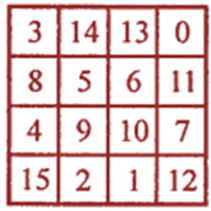3 + 8 + 4 = 15 + 15 = 30

3 + 12 = 5 + 10 = 30

3 + 14 + 0 = 17 + 13 = 30

0 + 6 = 9 + 15 = 30

0 + 7 + 12 = 19 + 11 =30

15 + 1 + 12 = 28 + 2 = 30

8 + 6 + 11 = 25 + 5 = 30

13 + 6 + 1 = 20 + 10 = 30

14 + 5 + 2 = 21 + 9 = 30

14. Fill in the blanks to complete the following number triangle: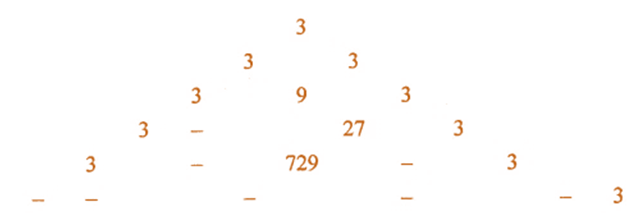Solution:

Here is the complete triangle: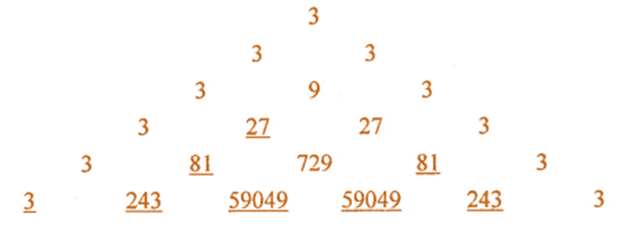EXERCISE 5.3

1. Which of the following numbers are divisible by 5 or by 10:
(i) 87035
(ii) 75060
(iii) 9685
(iv) 10730
Solution:

We know that,

A number is divisible by 5 if its unit digit is 5 or 0.

A number is divisible by 10 if its unit digit is 0.

So, 87035, 75060, 9685, 10730 are all divisible by 5.

75060 and 10730 are divisible by 10.

2. Which of the following numbers are divisible by 2, 4 or 8:
(i) 67894
(ii) 5673244
(iii) 9685048
(iv) 6533142
(v) 75379
Solution:

A number is divisible by 2 if its unit digit is 2, 4, 6, 8 or 0.

A number is divisible by 4 if the number formed by the last two digits is divisible by 4.

A number is divisible by 8 if the number formed by the last three digits is divisible by 8.

So Number 67894, 5673244, 9685048, 6533142 are divisible by 2.

Numbers, 5673244, 9685048 are divisible by 4

and numbers 9685048 is divisible by 8.

3. Which of the following numbers are divisible by 3 or 9:
(i) 45639
(ii) 301248
(iii) 567081
(iv) 345903
(v) 345046
Solution:

A number is divisible by 3 if the sum of its digits is divisible by 3.

A number is divisible by 9 if the sum of its digits is divisible by 9.

So the numbers 45639, 301248, 567081, 345903 are divisible by 3.

And 49639, 301248, 467081 are divisible by 9.

4. Which of the following numbers are divisible by 11:
(i) 10835
(ii) 380237
(iii) 504670
(iv) 28248
Solution:

A number is divisible by 11 if the difference of the sum of digits at the odd places and sum of the digits at even places is zero or divisible by 11.

So the numbers 10835, 380237, 28248 are divisible by 11.

5. Which of the following numbers are divisible by 6:
(i)15414
(ii) 213888
(iii) 469876
Solution:

A number is divisible by 6 if it is divisible by 2 as well as by 3.

So the numbers 15414 and 213888 are divisible by 6.

6. Which of the following numbers are divisible by 7:
(i) 46f8894875
(ii) 3794856
(iii) 39823
Solution:

A number is divisible by 7 if the difference of the sum of digits in alternate blocks of three digits from the right to the left is divisible by 7.

So the numbers 4618894875 and 39823 are divisible by 7.

7. (i) If 34x is a multiple of 3, where x is a digit, what is the value of x?
(ii) If 74 × 5284 is a multiple of 3, where x is a digit, find the value(s) of x.
Solution:

(i) 34x is a multiple of 3

If 3 + 4 + x = 7 + x is divisible by 3

Then x + 7 = 9

x = 9 – 7

= 2

∴ x = 2, 5, 8

(ii) 74 × 5284 is divisible by 3

7 + 4 + x + 5 + 2 + 8 + 4 is divisible by 3

Then, 30 + x is divisible by 3

∴ x = 0, 3, 6, 9

8. If 42z3 is a multiple of 9, where z is a digit, what is the value of z?
Solution:

42z3 is a multiple of 9

Then, 4 + 2 + z + 3 is divisible by 9

9 + z is divisible by 9

So either 9 + z = 9 or 9 + z = 0

z = 9 + 9 = 18, or z = 9 – 9 = 0

∴ z = 0, 9

9. In each of the following replace × by a digit so that the number formed is divisible by 9:
(i) 49 × 2207
(ii) 5938 × 623
Solution:

(i) 49 × 2207 is divisible by 9

Then, 4 + 9 + x + 2 + 2 + 0 + 7 is divisible by 9

24 + x is divisible by 9

24 + x = 27

x = 27 – 24

= 3, which is divisible by 9

∴ x = 3

(ii) 5938 × 623 is divisible by 9

Then, 5 + 9 + 3 + 8 + x + 6 + 2 + 3 is divisible by 9

36 + x is divisible by 9

So, 36 + x = 36 or 45

x = 36 – 36 = 0 or x = 45 – 36 = 9

∴ x = 0, 9

10. In each of the following replace * by a digit so that the number formed is divisible by 6:
(i) 97 × 542
(ii) 709 × 94
Solution:

(i) 97 × 542

It is divisible by 6

It is divisible by 2 and 3

Since its unit digit is 2

∴ It is divisible by 2.

It is divisible by 3

Since, its sum of its digits 9 + 7 + 5 + 4 + 2 = 27 [which is divisible by 3]

27 + ‘*’ = 27, or 30, 33, 36

∴ The ‘*’ place can be replaced by 0 or 3 or 6 or 9.

(ii) 709 × 94

It is divisible by 6

It is divisible by 2 and 3

We know that its unit digit is 4

∴ It is divisible by 2

It is divisible by 3

Since its sum of its digits = 7 + 0 + 9 + 9 + 4 + * = 29 + * [which is divisible by 3]
29 + * = 30, or 33, or 36

∴ The ‘*’ place can be replaced by 1 or 4 or 7.

11. In each of the following replace * by a digit so that the number formed is divisible by 11:
(i) 64 × 2456
(ii) 86 × 6194
Solution:

(i) 64 × 2456

It is divisible by 11

The difference between the sum of digits of odd places and sum of digits of even place is divisible by 11or it is zero.

Now, 6 + 4 + * + 6 – 5 + 2 + 4 [which is divisible by 11]
16 + * – 11 is divisible by 11

5 + x is divisible by 11

∴ * is 6.

(ii) 86 × 6194

It is divisible by 11

The difference between the sum of digits of odd places and sum of digits of even places is divisible by 11 or it is zero.

Now, 4 + 1 + * + 8 = 13 + *

9 + 6 + 6 = 21

21 – (13 + *) is divisible by 11

21 – 13 – * is divisible by 11

8 – * is divisible by 11

∴ * is 8.